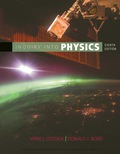# , Water flows straight down from an open faucet (Figure 4.55). The cross-sectional area of the faucet is 1.8 10-4 m a , and the speed of the water as it leaves me faucet is 0.85 m/s. Ignoring air resistance, find the cross-sectional area of the water stream at a point 0.10 m below the faucet opening.### Inquiry into Physics

8th Edition
Ostdiek
Publisher: Cengage
ISBN: 9781337515863### Inquiry into Physics

8th Edition
Ostdiek
Publisher: Cengage
ISBN: 9781337515863

#### Solutions

Chapter
Section
Chapter 4, Problem 14C
Textbook Problem
275 views

## , Water flows straight down from an open faucet (Figure 4.55). The cross-sectional area of the faucet is 1.8 10-4 ma, and the speed of the water as it leaves me faucet is 0.85 m/s. Ignoring air resistance, find the cross-sectional area of the water stream at a point 0.10 m below the faucet opening.

Expert Solution
To determine

The cross-sectional area of the water stream at a point 0.10m below the faucet opening.

### Explanation of Solution

Given info:

Area of faucet=0.000180m2

Speed of water = 0.85 m/s

Height=0.10m

Formula used:

Kinetic relations V2=Vi2+2gh.

Calculation:

Substitute the values in above equation

V22=V12+2gh

=(0.85)2+2(9.8)(0.10)

=2.6825

V=1.637

So the cross sectional area of the water at a point 0

### Want to see the full answer?

Check out a sample textbook solution.See solution

### Want to see this answer and more?

Bartleby provides explanations to thousands of textbook problems written by our experts, many with advanced degrees!

See solution